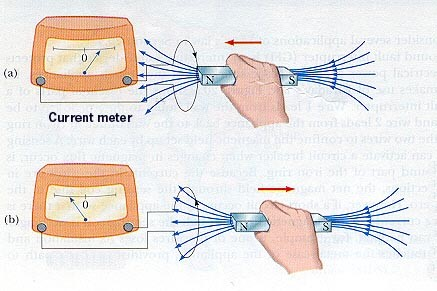Induction heating works because there is an alternating electrical current which is applied to the primary of a transformer, as that creates an alternating magnetic field. Faraday's Law states if the secondary of the transformer is located within the magnetic field, an electric current is induced. In this post, we'll dig into the theory behind Faraday's Law of Induction, as it's important to understanding the theory of induction heating.

Faraday’s Law of induction is a basic principle of electricity that describes how changing magnetic fields can cause a current to flow in conductors. In the figure above you can see a bar magnet being moved in and out of a wire loop. As the bar magnet is moved towards the loop it induces a current traveling clockwise through the wire circuit. When the magnet is moved away from the wire loop, the current in the loop switches direction and travels counter-clockwise.

Note how in the figure above both times a current is induced the bar magnet is in movement. This is a fundamental principle of Faraday’s Law. In order for a magnetic field to induce a current in a wire the strength of the magnetic flux through that wire must be changing. If the magnet were to be held in a stationary position no current would be induced in the wire.

Faraday’s Law outlined the mathematical formula used to describe the current induced for a changing flux. Since the mathematical formula requires the use of calculus, it will not be covered in this post. However, it is important to understand the basic principle of Faraday’s Law of Induction, which is why we covered it here.